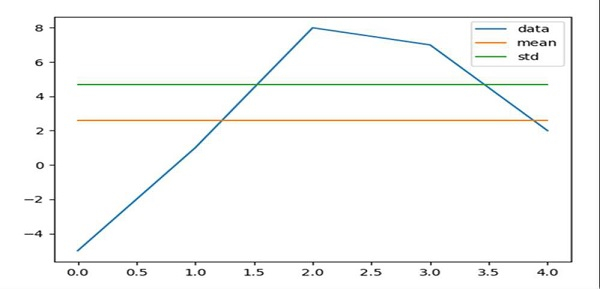# Plot mean and standard deviation in Matplotlib

First, we can calculate the mean and standard deviation of the input data using Pandas dataframe.

Then, we could plot the data using Matplotlib.

## Steps

• Create a list and store it in data.

• Using Pandas, create a data frame with data (step 1), mean, std.

• Plot using a dataframe.

• To show the figure, use plt.show() method.

## Example

import pandas as pd
from matplotlib import pyplot as plt

data = [-5, 1, 8, 7, 2]

df = pd.DataFrame({
'data': data,
'mean': [2.6 for i in range(len(data))],
'std': [4.673328578 for i in range(len(data))]})

df.plot()
plt.show()

## Output